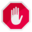## Name

ST_CollectionHomogenize — Returns the simplest representation of a geometry collection.

## Synopsis

`geometry ST_CollectionHomogenize(`geometry collection`)`;

## Description

Given a geometry collection, returns the "simplest" representation of the contents.

• Homogeneous (uniform) collections are returned as the appropriate multi-geometry.

• Heterogeneous (mixed) collections are flattened into a single GeometryCollection.

• Collections containing a single atomic element are returned as that element.

• Atomic geometries are returned unchanged. If required, these can be converted to a multi-geometry using ST_Multi.This function does not ensure that the result is valid. In particular, a collection containing adjacent or overlapping Polygons will create an invalid MultiPolygon. This situation can be checked with ST_IsValid and repaired with ST_MakeValid.

Availability: 2.0.0

## Examples

Single-element collection converted to an atomic geometry

```  SELECT ST_AsText(ST_CollectionHomogenize('GEOMETRYCOLLECTION(POINT(0 0))'));

st_astext
------------
POINT(0 0)
```

Nested single-element collection converted to an atomic geometry:

```SELECT ST_AsText(ST_CollectionHomogenize('GEOMETRYCOLLECTION(MULTIPOINT((0 0)))'));

st_astext
------------
POINT(0 0)
```

Collection converted to a multi-geometry:

```SELECT ST_AsText(ST_CollectionHomogenize('GEOMETRYCOLLECTION(POINT(0 0),POINT(1 1))'));

st_astext
---------------------
MULTIPOINT(0 0,1 1)
```

Nested heterogeneous collection flattened to a GeometryCollection:

```SELECT ST_AsText(ST_CollectionHomogenize('GEOMETRYCOLLECTION(POINT(0 0), GEOMETRYCOLLECTION( LINESTRING(1 1, 2 2)))'));

st_astext
---------------------
GEOMETRYCOLLECTION(POINT(0 0),LINESTRING(1 1,2 2))
```

Collection of Polygons converted to an (invalid) MultiPolygon:

```SELECT ST_AsText(ST_CollectionHomogenize('GEOMETRYCOLLECTION (POLYGON ((10 50, 50 50, 50 10, 10 10, 10 50)), POLYGON ((90 50, 90 10, 50 10, 50 50, 90 50)))'));

st_astext
---------------------
MULTIPOLYGON(((10 50,50 50,50 10,10 10,10 50)),((90 50,90 10,50 10,50 50,90 50)))
```